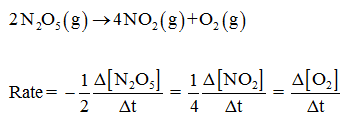# The decomposition of dinitrogen pentoxide is described by the chemical equation 2 N2O5(g) 4 NO2(g) + O2(g)If the rate of disappearance of N2O5 is equal to 0.60 mol/min, what is the rate of appearance of O2?A. 4.8 mol/minB. 0.30 mol/minC. 1.2 mol/minD. 2.4 mol/min

Question
18 views

The decomposition of dinitrogen pentoxide is described by the chemical equation 2 N2O5(g) 4 NO2(g) + O2(g)

If the rate of disappearance of N2O5 is equal to 0.60 mol/min, what is the rate of appearance of O2?

A. 4.8 mol/min

B. 0.30 mol/min

C. 1.2 mol/min

D. 2.4 mol/min

check_circle

Step 1

The balanced equation and the rate of the equation is expressed below:...

### Want to see the full answer?

See Solution

#### Want to see this answer and more?

Solutions are written by subject experts who are available 24/7. Questions are typically answered within 1 hour.*

See Solution
*Response times may vary by subject and question.
Tagged in